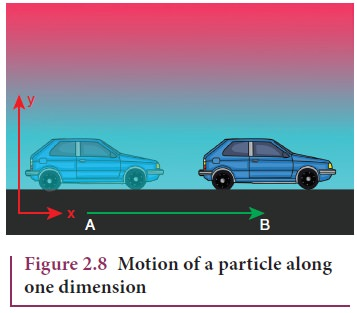Home | | Physics 11th std | Motion in One, Two and Three Dimensions

# Motion in One, Two and Three Dimensions

Let the position of a particle in space be expressed in terms of rectangular coordinates x, y and z.

Motion in One, Two and Three Dimensions

Let the position of a particle in space be expressed in terms of rectangular coordinates x, y and z. When these coordinates change with time, then the particle is said to be in motion. However, it is not necessary that all the three coordinates should together change with time. Even if one or two coordinates changes with time, the particle is said to be in motion. Then we have the following classification.

## i. Motion in one dimension

One dimensional motion is the motion of a particle moving along a straight line.

This motion is sometimes known as rectilinear or linear motion.

In this motion, only one of the three rectangular coordinates specifying the position of the object changes with time.

For example, if a car moves from position A to position B along x-direction, as shown in Figure 2.8, then the variation in x-coordinate alone is noticed.### Examples

·           Motion of a train along a straight railway track.

·           An object falling freely under gravity close to Earth.

## ii. Motion in two dimensions

If a particle is moving along a curved path in a plane, then it is said to be in two dimensional motion.

In this motion, two of the three rectangular coordinates specifying the position of object change with time.

For instance, when a particle is moving in the y - z plane, x does not vary, but y and z vary as shown in Figure 2.9### Examples

·           Motion of a coin on a carrom board.

·           An insect crawling over the floor of a room.

## iii. Motion in three dimensions

A particle moving in usual three dimensional space has three dimensional motion.

In this motion, all the three coordinates specifying the position of an object change with respect to time. When a particle moves in three dimensions, all the three coordinates x, y and z will vary.

### Examples

·           A bird flying in the sky.

·           Random motion of a gas molecule.

·           Flying of a kite on a windy day.

Study Material, Lecturing Notes, Assignment, Reference, Wiki description explanation, brief detail
11th Physics : UNIT 2 : Kinematics : Motion in One, Two and Three Dimensions |